# Exercise 11.3 Perimeter-and-Area -NCERT Solutions Class 7

Go back to  'Perimeter and Area'

## Chapter 11 Ex.11.3 Question 1

Find the circumference of the circles with the following radius:

\begin{align}\left( {{\rm{Take}}\;\pi = \frac{{22}}{7}} \right)\end{align}

(a) $$14 \,\rm cm$$

(b) $$28 \,\rm mm$$

(c) $$21 \,\rm cm$$

### Solution

What is known?

What is unknown?

The circumference of the circles.

Reasoning:

In this question, radius of the circle is given. Put the value of the radius in the formula of circumference of the circle to find out the circumference.

Steps:

(a) Circumference of the circle

\begin{align} &= 2\pi r\\&= 2 \times \frac{{22}}{7} \times 14\\&= 2 \times 22 \times 2\\&= 88\;\rm{cm}\end{align}

(b) Circumference of the circle

\begin{align}&= 2\pi r\\&= 2 \times \frac{{22}}{7} \times 28\\&= 2 \times 22 \times 4\\&= 176\;\rm{mm}\end{align}

(c) Circumference of the circle

\begin{align}&= 2\pi r\\&= 2 \times \frac{{22}}{7} \times 21\\&= 2 \times 22 \times 3\\&= 132\;\rm{cm}\end{align}

## Chapter 11 Ex.11.3 Question 2

Find the area of the following circles, given that:\begin{align}\left( {{\rm{Take\, }}\pi = \frac{{22}}{7}} \right)\end{align}

(a) Radius $$= 14\,\rm mm$$

(b) Diameter $$= 49 \,\rm m$$

(c) Radius $$= 5 \,\rm cm$$

### Solution

What is known?

Radius or diameter of the circles.

What is unknown?

The Area of the circles.

Reasoning:

In this question, radius (or diameter) of the circle is given. Put the value of the radius in the formula of area of the circle to find out the area.

Steps:

(a) Given,

Radius of the circle $$= 14 \,\rm mm$$

Area of circle

\begin{align}&= \pi {r^2}\\&= \frac{{22}}{7} \times 14 \times 14\\&= 22 \times 2 \times 14\\&= 616\;\rm m{m^2}\end{align}

(b) Given,

Diameter of the circle $$= 49 \,\rm m$$

Therefore, radius $$= 24.5 \,\rm m$$

Area of circle

\begin{align}&= \pi {r^2}\\&= \frac{{22}}{7} \times 24.5 \times 24.5\\&= 22 \times 24.5 \times 3.5\\&= 1886.5\;\rm {m^2}\end{align}

(c) Given,

Radius of the circle $$= 5 \,\rm cm$$

Area of circle

\begin{align}&= \pi {r^2}\\&= \frac{{22}}{7} \times 5 \times 5\\ &= \frac{{550}}{7}\\&= 78.57\;\rm c{m^2}\end{align}

## Chapter 11 Ex.11.3 Question 3

If the circumference of a circular sheet is $$154\,\rm m$$, find its radius. Also find the area of the sheet. \begin{align}\left( {{\rm{Take }}\pi = \frac{{22}}{7}} \right)\end{align}

### Solution

What is known?

The circumference of a circular sheet is $$154\,\rm m$$.

What is unknown?

Radius and the area of the circular sheet.

Reasoning:

This question is straight forward, circumference of a circular sheet is given as $$154\,\rm m$$ and it will also be equal to the formula of circumference of the circle, equate them and find the value of unknown i.e. radius. Now, the radius is known put the value in the formula of area of circle and find the measurement of the area.

Steps:

Circumference of the circular sheet$$= 154 m$$

\begin{align}2\pi r &= 154\\2 \times \frac{{22}}{7} \times r &= 154\\r &= \frac{{154 \times 7}}{{2 \times 22}}\\r &= \frac{{49}}{2}\\r &= 24.5\;\rm{m}\end{align}

Now,

Area of circular sheet

\begin{align} &= \pi {r^2}\\&= \frac{{22}}{7} \times 24.5 \times 24.5\\&= 1886.5\;\rm{m^2}\end{align}

Thus, the radius and area of circular sheet are $$24.5 \rm \,m^2$$ and $$1886.5 \rm\,m^2$$ respectively.

## Chapter 11 Ex.11.3 Question 4

A gardener wants to fence a circular garden of diameter $$21\rm\,m.$$ Find the length of the rope he needs to purchase, if he makes $$2$$ rounds of fence. Also find the cost of the rope,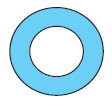if it costs $$\rm{Rs}\,4$$ per meter.

\begin{align}\left( {{\rm{Take }}\pi = \frac{{22}}{7}} \right)\end{align}

### Solution

What is known?

Diameter of circular garden and the cost of one meter of rope.

What is unknown?

The length of the rope required if the gardener wants to make $$2$$ rounds of fence and also the cost of the rope, if it costs ₹ $$4$$ per meter.

Reasoning:

As the diameter is given as $$21 \rm\,m$$ that means the radius will be the half of the diameter. Using the diameter, circumference of the circular garden can be calculated. Since the gardener wants to make $$2$$ rounds of fence, the length of rope required will be two time the circumference. The cost of the rope can be obtained by multiplying length of the rope with cost of one meter of the rope.

Steps:

Diameter of the circular garden

$$= 21 \rm\,m$$

\begin{align}=\frac{{21}}{2}\,\rm{m}\end{align}

Now,

Circumference of circular garden

\begin{align}&= 2\pi r\\ &= 2 \times \frac{{22}}{7} \times \frac{{21}}{2}\\&= 66\;\rm{m}\end{align}

Since, the gardener wants to make $$2$$ rounds of fence, so the total length of the rope required for fencing $$= 2 \,\times \, 66 = 132\rm\, m$$

Since, the cost of $$1$$-meter rope $$= ₹ \,4$$

Therefore, the cost of $$132$$-meter rope $$= 4 \times 132 = ₹\, 528$$

## Chapter 11 Ex.11.3 Question 5

From a circular sheet of radius $$4 \rm\,cm$$, a circle of radius $$3\rm\, cm$$ is removed. Find the area of the remaining sheet. \begin{align}(\text{Take}\, π = 3.14)\end{align}

### Solution

What is known?

Radius of circular sheet from which a circle of radius $$3\rm\, cm$$ is removed.

What is the unknown?

The area of the remaining sheet.

Reasoning:

To find the area of the remaining sheet, first find the area of both the circular sheets and then subtract the area of circle sheet of radius $$3\rm\, cm$$ from the area of circular sheet of radius $$4 \rm\,cm$$.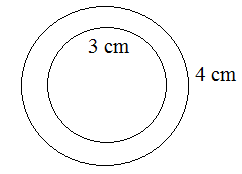Steps:

Radius of circular sheet (r1) $$=$$ $$4 \rm\,cm$$

Radius of removed circle (r2) $$=$$ $$3\rm\, cm$$

Area of remaining sheet $$=$$ Area of circular sheet $$-$$ Area of removed circle

\begin{align} &= \pi {r_1}^2 - \pi {r_2}^2\\ &= \pi \left( {{r_1}^2 - {r_2}^2} \right)\\ &= 3.14{\rm{ }}\left[ {\left( {{4^2}} \right) - \left( {{3^2}} \right)} \right]\\ &= 3.14\left( {16 - 9} \right)\\ &= 3.14 \times 7\\ &= 21.98{\rm{ }}\rm\,c{m^2}\end{align}

Thus, the area of remaining sheet is $$21.98 \rm\,cm^2.$$

## Chapter 11 Ex.11.3 Question 6

Saima wants to put a lace on the edge of a circular table cover of diameter $$1.5 \rm\,m.$$ Find the length of the lace required and also, find its cost if one meter of the lace costs $$\rm Rs. \,15.$$ (Take $$π = 3.14$$)

### Solution

What is known?

Diameter of the circular table and cost of one meter of lace.

What is unknown?

The length of the lace required and the overall cost of lace if the cost of one meter of the lace is $$₹\,15.$$

Reasoning:

Since Saima wants to put lace on the edge of the circular table cover, that means length of the lace will be equal to the circumference of circular table cover. Find circumference of circle using the radius of the circular table cover. Now, to find the overall cost of lace multiply the total length of lace by$$₹\,15.$$

Steps:

Given, Diameter of the circular table cover

$$= 1.5 \rm\,m$$

Radius of the circular table cover

\begin{align}=\frac{1}{2}\end{align} \rm \,m = 0.75\rm\, m

Circumference of circular table cover

\begin{align}&= 2\pi r\\&= 2 \times 3.14 \times 0.75\\ &= 4.71\end{align}

Therefore, the length of required lace is $$4.71 \rm\,m.$$

Since the cost of $$1\rm\, m$$ lace is $$₹ \,15$$

Therefore, the cost of $$4.71 \rm\,m$$ lace

$$= ₹\,15\, \times\, 4.71 = ₹ \,70.65$$

## Chapter 11 Ex.11.3 Question 7

Find the perimeter of the adjoining figure, which is a semicircle including its diameter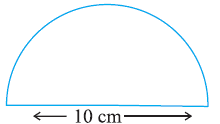### Solution

What is known?

Diameter of the semicircle.

What is unknown?

The perimeter of the semicircle including its diameter.

Reasoning:

To find the perimeter of the given figure, add the circumference or perimeter of the semi-circle and the diameter of the given figure.

Steps:

Given, diameter $$= 10 \rm\,cm$$

Radius \begin{align}=\frac{{10}}{2}\end{align}= 5 \rm\,cm

Perimeter of the given figure $$=$$ Circumference of semi $$-$$ circle $$+$$ diameter

\begin{align}&= \frac{{2\pi r}}{2} + {\text{D}} \\{\text{ }}&= \pi r + {\text{D}} \\&= \frac{{22}}{7} \times 5 + 5 \\&= \frac{{110}}{7} + 5 \\&= 25.71\;\rm cm\end{align}

Thus, the perimeter of the given figure is $$25.71\rm\, cm.$$

## Chapter 11 Ex.11.3 Question 8

Find the cost of polishing a circular table-top of diameter $$1.6\rm\, m,$$ if the rate of polishing is $$\rm Rs.\, 15/\rm\,m^2.$$ (Take $$π = 3.14$$)

### Solution

What is known?

Diameter of the circular table-top and the rate of polishing per meter.

What is unknown?

The cost of polishing a circular tabletop.

Reasoning:

To find the cost of polishing, first find out the area of the tabletop. To find the total cost of polishing, multiply the total area by rate of polishing per meter $$(\rm Rs \,15).$$

Steps:

Diameter of the circular tabletop $$= 1.6\rm\, m$$

\begin{align}=\frac{{1.6}}{2}\end{align}$$= 0.8\rm\, m$$

Area of circular table top

\begin{align}&=\pi {r^2}\\ &= 3.14 \times 0.8 \times 0.8\\ &= 2.0096\;\rm{m^2}\end{align}

Since, the cost of $$1\,\rm m^2$$ polishing $$= ₹\,15$$

$$\therefore$$ the cost of polishing

$$2.0096 \rm\,m^2 = 15 \times 2.0096 = ₹ \,30.14$$

Thus, the cost of polishing a circular tabletop is $$₹ \,30.14$$

## Chapter 11 Ex.11.3 Question 9

Shazli took a wire of length $$44 \rm\,cm$$ and bent it into the shape of a circle. Find the radius of that circle. Also find its area. If the same wire is bent into the shape of a square, what will be the length of each of its sides? Which figure encloses more area, the circle or the square? \begin{align}\left( {{\text{Take }}\pi = \frac{{22}}{7}} \right)\end{align}

### Solution

What is known?

A wire of length $$44 \rm\,cm$$ which is bent into the shape of a circle and the same wire is bent into the shape of a square.

What is unknown?

The radius and area of that circle fotexted by bending a wire of length $$44 \rm\,cm$$ into the shape of a circle. If the same wire is bent into the shape of a square, what will be the length of each of its sides and which figure encloses more area, the circle or the square?

Reasoning:

Since wire of $$44 \rm\,cm$$ is bent in the fotext of circle, it means the circumference of the circle fotexted is $$44\rm\,m.$$ By using the fotextula of circumference of the circle you can find out the radius and area of circle. Next the same wire is bent in the fotext of square, which means that the perimeter of square will be $$44 \rm\,cm$$. From the perimeter, measure of each side of square can be calculated. Now you have areas of both the figures, and you can compare them to find which figure encloses more area.

Steps:

Given,

Length of the wire $$=$$ $$44 \,\rm cm$$

$$\therefore$$ the circumference of the circle is $$44 \,\rm cm$$

\begin{align}C &= 2\pi r\\44 &= 2\pi r\\44 &= 2 \times \frac{{22}}{7} \times r\\r &= \frac{{44 \times 7}}{{44}}\\r &= 7\;\rm{cm}\end{align}

Now,

Area of the circle

\begin{align} &= \pi {r^2} \\&= \frac{{22}}{7} \times 7 \times 7\\ &= 154\;\rm{cm^2}\end{align}

Now the wire is bent into square.Then,

Perimeter of square $$=44\, cm$$

\begin{align}4 \times {\rm{Side}} &= 44\\{\rm{Side}} &= \frac{{44}}{4} \\&= 11\;\rm{cm}\end{align}

Now,

Area of square

\begin{align} &= {\rm{Side}} \times {\rm{Side}}\\&= \;{\rm{11}} \times {\rm{11}}\\&= 121\,{\rm{c}}{{\rm{m}}^2}\end{align}

On comparing both the areas, it is clear that the area of circle is greater than that of square, so the circle enclosed more area.

## Chapter 11 Ex.11.3 Question 10

From a circular card sheet of radius $$14 \rm\,cm$$, two circles of radius $$3.5\rm\, cm$$ and a rectangle of length $$3\rm\, cm$$ and breadth $$1 \rm\,cm$$ are removed. (as shown in the adjoining figure). Find the area of the remaining sheet. (Take $$π =22/7$$ )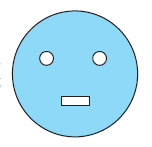### Solution

What is known?

From a circular card sheet of radius $$14 \rm\,cm$$, two circles of radius $$3.5\rm\, cm$$ and a rectangle of length $$3\rm\, cm$$ and breadth $$1 \rm\,cm$$ are removed.

What is unknown?

The area of the remaining sheet.

Reasoning:

From a circular card sheet of radius $$14 \rm\,cm$$,two circles of radius $$3.5\rm\, cm$$ and a rectangle of length $$3\rm\, cm$$ and breadth $$1 \rm\,cm$$ are removed. To find the area of the remaining card sheet, first find the area of the circular sheet then the area of the two circles of radius $$3.5\rm\, cm$$ and a rectangle of length $$3\rm\, cm$$ and breadth $$1 \rm\,cm$$. Then subtract the area of the three figures from the area of the circular sheet to get the area of the remaining sheet.

Steps:

Radius of circular sheet $$(R)$$ $$=$$ $$14 \rm\,cm$$ and radius of smaller circle (r) $$=$$ $$3.5\rm\, cm$$

Length of rectangle $$(l)$$ $$=$$ $$3\rm\, cm$$ and breadth of rectangle (b) $$=$$ $$1 \rm\,cm$$

Area of the remaining sheet = Area of circular sheet - Area of two smaller circle + Area of rectangle

\begin{align}&=\! \pi {R^2} \!-\! \left[ {2\pi {r^2} \!+\! \left( {{\rm{Length}} \!\times\! {\rm{Breadth}}} \right)} \right]\\ &=\! \frac{{22}}{7} \!\times\! 14 \!\times\! 14\! - \!\left[ {2 \!\times\! \frac{{22}}{7} \!\times\! 3.5 \!\times\! 3.5 \!+\! \left( {3 \!\times\! 1} \right)} \right]\\ &=\! 22 \!\times\! 2 \!\times\! 14\! -\! \left[ {\frac{{44}}{7} \!\times\! 3.5 \!\times\! 3.5 \!+\! 3} \right]\\ &\!= 616 \!-\! \left( {22 \!\times\! 3.5 \!+\! 3} \right)\\ &=\! 616 \!-\! \left( {77 \!+\! 3} \right)\\ &=\! 616 \!- \!80\\ &= \!536\;\rm c{m^2}\end{align}

Therefore, the area of remaining sheet is $$536 \rm\,cm^2.$$

## Chapter 11 Ex.11.3 Question 11

A circle of radius $$2\rm\, cm$$ is cut out from a square piece of an aluminum sheet of side $$6\rm\, cm$$. What is the area of the left-over aluminum sheet? (Take $$π = 3.14$$)

### Solution

What is known?

A square piece of an aluminum sheet of side $$6\rm\, cm$$ from which a circle of radius $$2\rm\, cm$$ is cut out.

What is unknown?

The area of the left-over aluminum sheet.

Reasoning:

First, find the area of the piece of the aluminum sheet then subtract the area of the circle from it and you can get the area of the left -over aluminum sheet.

Steps:

Side of square piece of aluminum sheet $$=$$ $$6\rm\, cm$$ and radius of circle $$=$$ $$2\rm\, cm$$

Area of aluminium sheet left $$=$$ Total area of square aluminum sheet $$-$$ Area of circle

\begin{align} &= {\rm{Side}} \times {\rm{Side}} - \pi {r^2}\\ &= 6 \times 6 - 3.14 \times 2 \times 2\\ &= 36 - 12.56\\ &= 23.44\;\rm c{m^2}\end{align}

Therefore, the area of aluminum sheet left is $$23.44 \rm\,cm^2.$$

## Chapter 11 Ex.11.3 Question 12

The circumference of a circle is $$31.4 \rm\,cm$$. Find the radius and the area of the circle? (Take $$π = 3.14$$)

### Solution

What is given ?

The circumference of a circle is $$31.4 \rm\,cm$$

What is the unknown ?

The radius and the area of the circle

Reasoning:

The circumference of the circle is given as $$31.4 \rm\,cm$$, using which you can find out the radius of the circle. Now the radius is known, put it in the formula of area of circle to obtain area of the circle.

Steps:

Given,

The circumference of the circle $$=31.4\,cm$$

\begin{align} 2\pi r &= 31.4\\2 \times 3.14 \times r &= 31.4\\r &= \frac{{31.4}}{{6.28}}\\r &= 5\;\rm cm\end{align}

Then,

Area of the circle

\begin{align} &= \pi {r^2}\\ &= 3.14 \times 5 \times 5\\ &= 78.5\;\rm c{m^2}\end{align}

Therefore, the radius and the area of the circle are $$5 \rm\,cm$$ and $$78.5 \rm\,cm^2$$ respectively.

## Chapter 11 Ex.11.3 Question 13

A circular flower bed is surrounded by a path $$4 \rm\,m$$ wide. The diameter of the flower bed is $$66 \rm\,m$$. What is the area of this path? $$(π = 3.14)$$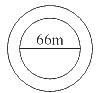### Solution

What is known?

A circular flower bed of diameter $$66 \rm\,m$$ which is surrounded by a path $$4 \rm\,m$$ wide.

What is unknown?

The area of the path.

Reasoning:

Since the diameter of the circular flower bed is $$66\rm\,m,$$ so, the radius of the circular bed will be $$33\rm\,m$$. The radius of the outer circle, which includes radius of the circular path as well width of the path is $$33\rm\,m + 4\rm\,m = 37\rm\,m$$. Now the areas of the inner circle of radius $$33\rm\,m$$ and outer circle of radius $$37 \rm\,m$$ can be calculated and by subtracting area of the inner circle from the area of the outer circle you will get the area of this path.

Steps:

Diameter of the circular flower bed $$=$$ $$66 \rm\,m$$

$$\therefore$$ Radius of circular flower bed (r) $$=$$ \begin{align}\frac{{66}}{2}\end{align} $$= 33 \rm\,m$$

$$\therefore$$ Radius of circular flower bed with $$4 \rm\,m$$ wide path $$(R) = 33 + 4 = 37 \rm\,m$$

Area of path = Area of bigger circle-Area of smaller circle

\begin{align}&=\pi {{\rm{R}}^2} - \pi {r^2}\\&= \pi \left( {{{\rm{R}}^2} - {r^2}} \right)\\ &= 3.14\left[ {{{\left( {37} \right)}^2} - {{\left( {33} \right)}^2}} \right]\\ &= 3.14\left [{\left({37 + 33} \right)\left( {37-33} \right)} \right] \end{align}

Using this formula,

\begin{align} (a^2 -b^2)= [( {a + b} ) ( {a - b} )]\end{align}

\begin{align} & = 3.14 \times 70 \times 4\\ &= 879.20\,\rm {m^2}\end{align}

Therefore, the area of the path is $$879.20 \rm\,m^2.$$

## Chapter 11 Ex.11.3 Question 14

A circular flower garden has an area of $$314\rm\, m^2$$. A sprinkler at the centre of the garden can cover an area that has a radius of $$12\rm\, m.$$ Will the sprinkler can water the entire garden? (Take $$π = 3.14$$).

### Solution

What is known?

A circular flower garden with an area of $$314\rm\, m^2$$ and a sprinkler at the centre of the garden can cover an area that has a radius of $$12\rm\, m$$

What is the unknown?

Will the sprinkler water the entire garden?

Reasoning:

A circular garden has area of $$314\rm\, m^2$$ and there is a sprinkler at the center of the garden which can water the area of radius $$12\rm\,m.$$ So, first we have to find the area covered by the sprinkler. Compare the area covered by the sprinkler and the area of the garden. If the area covered by sprinkler is more that means it will sprinkle the entire garden.

Steps:

Area covered by the sprinkler

\begin{align} &= 2\pi r \\&= 3.14 \times 12 \times 12\\&= 452.16{\rm{ }}\rm\,{m^2}\end{align}

Area of the circular flower garden $$= 314\rm\, m^2$$

Since Area of circular flower garden is smaller than area covered by the sprinkler, the sprinkler will water the entire garden.

## Chapter 11 Ex.11.3 Question 15

Find the circumference of the inner and the outer circles, shown in the adjoining figure? (Take $$π = 3.14$$)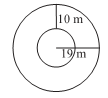### Solution

What is known?

Inner and outer circles of radius $$10 \rm\,m$$ and 1$$9 \rm\,m$$.

What is unknown?

The circumference of the inner and the outer circles.

Reasoning:

This question is straight forward, use the given information as the inner and outer radius of the circle is given $$10 \rm\,m$$ and 1$$9 \rm\,m$$, by using formula of the circumference of the circle you can find out the circumference of the inner and outer circles.

Steps:

Radius of the outer circle $$(r_1) = 19 \rm\,m$$

Therefore,

Circumference of outer circle

\begin{align}&= 2\pi {r_1} \\&= 2 \times 3.14 \times 19\\ &= 119.32{\rm{ }}\rm\,m\end{align}

Radius of inner circle$$(r_2)$$

\begin{align} &= 19 \,– 10 \\&= 9\rm\, m\end{align}

Therefore,

Circumference of outer circle

\begin{align} &= 2\pi {r_2}\\ &= 2 \times 3.14 \times 9\\ &= 56.52{\rm{ }}\rm \,m\end{align}

Therefore, the circumferences of inner and outer circles are $$56.52 \rm\,m$$ and $$119.32 \rm\,m$$ respectively.

## Chapter 11 Ex.11.3 Question 16

How many times a wheel of radius $$28 \rm\,cm$$ must rotate to go $$352 \rm\,m$$? \begin{align}\left( {{\text{Take }}\pi = \frac{{22}}{7}} \right)\end{align}

### Solution

What is known?

A wheel of $$28 \rm\,cm$$.

What is unknown?

Number of times the given wheel must rotate to go $$352 \rm\,m$$.

Reasoning:

The distance covered by the wheel in one revolution is equal to the circumference of the wheel. Now, to find out the number of times wheel should rotate, divide the total distance covered by the circumference of the wheel.

Steps:

Radius of wheel $$=$$ $$28 \rm\,cm$$

Circumference of wheel

\begin{align} &= 2\pi r \\ &= 2 \times \frac{{22}}{7} \times 28\\ &= 2 \times 22 \times 4\\ &= 176\;\rm\,cm\end{align}

Total distance $$=$$ $$352 \rm\,m$$ $$= 35200 \rm\,cm$$

No of times wheel should rotate

\begin{align} &= \frac{\text{Total distance covered by wheel}}{\text{Circumference of the wheel}}\\&= \frac{{35200}}{{176}}\\&= 200\end{align}

Therefore, wheel must rotate $$200$$ times to go $$352 \rm\,m$$.

## Chapter 11 Ex.11.3 Question 17

The minute hand of a circular clock is $$15 \rm\,cm$$ long. How far does the tip of the minute hand move in $$1$$ hour? (Take $$π = 3.14$$)

### Solution

What is known?

The minute hand of a circular clock is $$15 \rm\,cm$$ long.

What is unknown?

Distance covered by tip of minute hand in $$1$$ hour.

Reasoning:

In one hour, the minute hand completes one full circle. So, the distance covered by tip is equal to the circumference of the circle.

Steps:

Radius of the circle $$(r) =$$ $$15 \rm\,cm$$

Circumference of circular clock

\begin{align} &= 2\pi r \\ &= 2 \times 3.14 \times 15\\ &= {\rm{ }}94.2{\rm{ }}\rm\,cm\end{align}

Therefore, the tip of the minute hand moves $$94.2 \rm\,cm$$ in $$1$$ hour.

Related Sections
Related Sections
Instant doubt clearing with Cuemath Advanced Math Program